This lesson demonstrates that the exact balance between the effects of SPM and GVD leads to the formation of a fundamental soliton – a light pulse that propagates without changing its shape and spectrum, and shows some basic features of the higher-order solitons. Figure 1 shows the layout and its global parameters.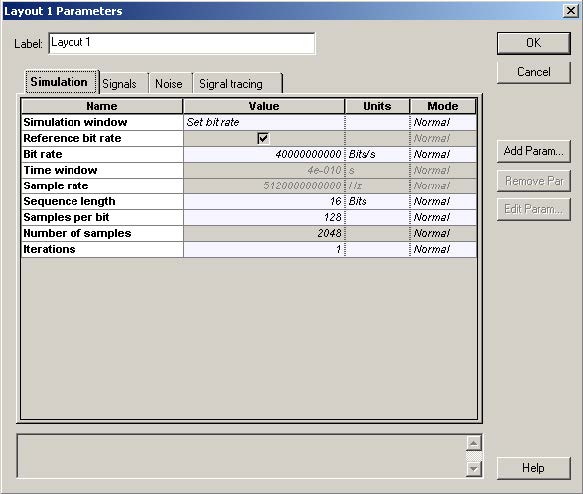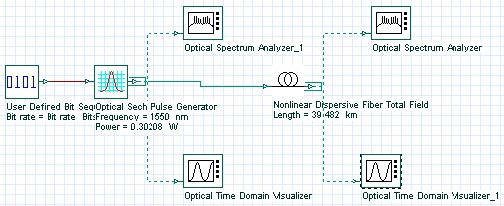Figure 1: System layout and global parameters

The compensation between the effects of SPM and GVD is not complete for Gaussian pulses since the SPM induced chirp is different from that induced by the GVD. The exact compensation occurs when the pulse shape is that of a fundamental soliton. The peak power necessary to launch a N-order soliton can be calculated in the following way .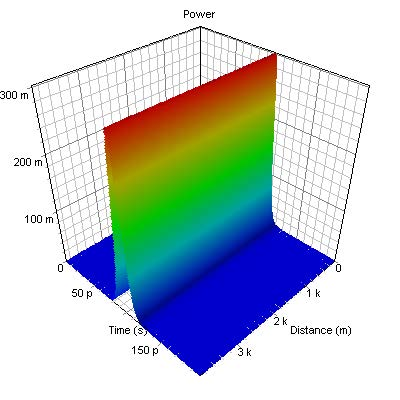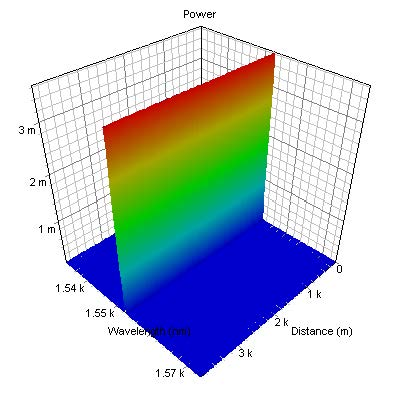Figure 2: Evolution of pulse shape (top) and spectrum (bottom) corresponding to the fundamental (N=1) soliton. One soliton period is shown.

At 40Gb/s the bit slot is 25 ps and the pulse width is TFWHM = 12.5ps.

The relation between the T0 parameter in (2) and TFWHM for sech-pulses is: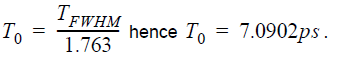The values n2 = 2.6X10-20m2 /W and aeff = 80 &mu;m2 are used.

The power value is:. .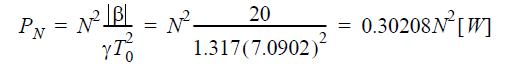The dispersion length is.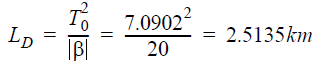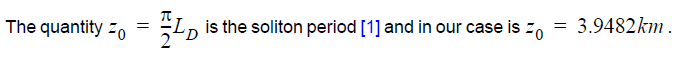Figure 2 shows the evolution of a fundamental soliton within one soliton period. The fundamental soliton remains unaffected by both SPM and GVD, since in this case they cancel each other completely. The pulse remains chirpless, due to the exact compensation that occurs between the SPM-induced and GVD-induced frequency modulations.

While the fundamental (N=1) soliton does not change its shape and spectrum with propagation the evolution of all higher order solitons (N>1) is spatially periodic with the period equal to z0. Figures 3 and 4 represent the evolution of pulse shape and spectrum corresponding to second-order (N=2) and third-order (N=3) solitons.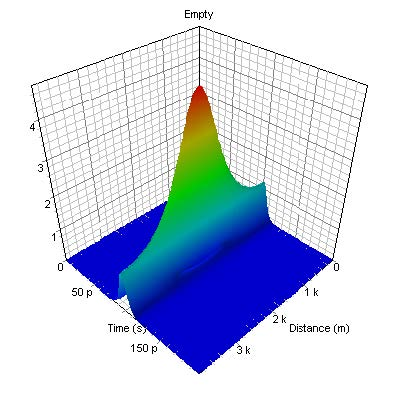Figure 3: Evolution of pulse shape (first plot) and spectrum (second plot) corresponding to a second-order soliton. One soliton period is shown.

After one complete period initial pulse shape is restored. The evolution of the spectrum also follows a periodic pattern.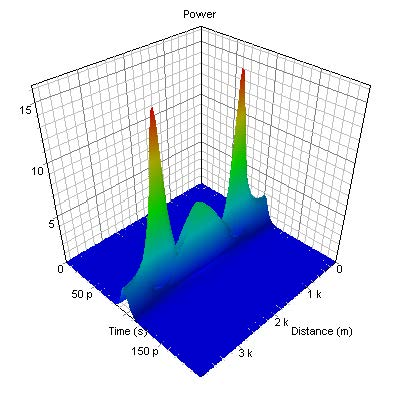Figure 4:  Evolution of N=3 soliton over one soliton period

Reference
  G. P. Agrawal Nonlinear Fiber Optics, Academic Press (2001).